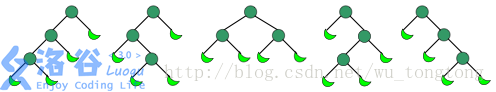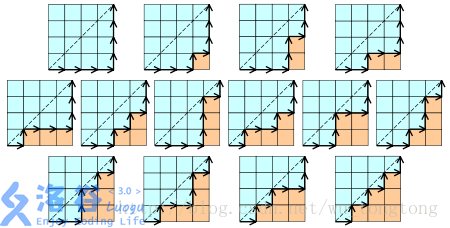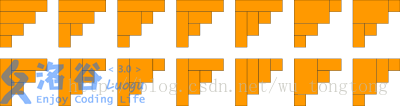## 公式

$Cat_{n}=\frac{1}{n+1}C_{2n}^{n}=\frac{(2n)!}{(n+1)!n!}$

$Cat_{n}=\frac{4n+2}{n+1}Cat_{n-1}$

$Cat_{n}=C_{2n}^{n}-C_{2n}^{n-1}$

## 实际问题的解决

### 经典问题：

#### 一

Dyck word

111000 101100 101010 110010 110100

$Cat_n=C_{2n}^{n}-C_{2n}^{n+1}=\frac{1}{n+1}C_{2n}^{n}$

#### 三

$Cat_n$表示有$n+1$个叶子的二叉树的个数#### 四

$Cat_n$表示所有不同构的含$n$个分枝结点的满二叉树的个数

（一个有根二叉树是满的当且仅当每个结点都有两个子树或没有子树）

#### 五

$Cat_n$表示所有在$n × n$格点中不越过对角线的单调路径的个数 一个单调路径从格点左下角出发，在格点右上角结束，每一步均为向上或向右#### 六

$Cat_n$表示通过连结顶点而将$n + 2$边的凸多边形分成三角形的方法个数 下图中为$n = 4$的情况：#### 七

$Cat_n$表示对{$1, …, n$}依序进出栈的置换个数 一个置换w是依序进出栈的当$S(w) = (1, …, n)$, 其中$S(w)$递归定义如下：令$w = unv$，其中$n$为$w$的最大元素，$u$和$v$为更短的数列 再令$S(w)$ =$S(u)S(v)n$，其中S为所有含一个元素的数列的单位元。

#### 八

$Cat_n$表示集合{$1, …, n$}的不交叉划分的个数. 其中每个段落的长度为$2$

#### 九

$Cat_n$表示用$n$个长方形填充一个高度为$n$的阶梯状图形的方法个数 下图为 $n = 4$的情况:## 应用总结

（实质上却都一样，无非是递归等式的应用，就看你能不能分解问题写出递归式了）

### 1.括号化问题

矩阵链乘： $P=a1×a2×a3×……×an$，依据乘法结合律，不改变其顺序，只用括号表示成对的乘积，试问有几种括号化的方案？($h(n)$种)

### 2.出栈次序问题。

一个栈(无穷大)的进栈序列为$1,2,3,..n$,有多少个不同的出栈序列?

类似：

(1)有$2n$个人排成一行进入剧场。入场费$5$元。其中只有$n$个人有一张$5$元钞票，另外$n$人只有$10$元钞票，剧院无其它钞票，问有多少中方法使得只要有$10$元的人买票，售票处就有$5$元的钞票找零？(将持$5$元者到达视作将5元入栈，持$10$元者到达视作使栈中某$5$元出栈)

(2)在圆上选择$2n$个点,将这些点成对连接起来，使得所得到的$n$条线段不相交的方法数。

### 3.将多边行划分为三角形问题

将一个凸多边形区域分成三角形区域的方法数?

类似：一位大城市的律师在她住所以北$n$个街区和以东$n$个街区处工作。每天她走$2n$个街区去上班。如果她从不穿越（但可以碰到）从家到办公室的对角线，那么有多少条可能的道路？

类似：在圆上选择$2n$个点,将这些点成对连接起来使得所得到的$n$条线段不相交的方法数?

### 4.给顶节点组成二叉树的问题。

给定$N$个节点，能构成多少种形状不同的二叉树？

先去一个点作为顶点,然后左边依次可以取0至N-1个相对应的,右边是N-1到0个,两两配对相乘,就是$h(0)*h(n-1) + h(2)*h(n-2) +…+ h(n-1)h(0)=h(n)$（能构成$h(N)$个）